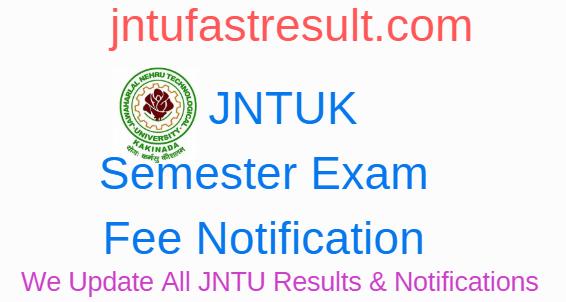# JNTUK All Courses Exam Fee Details – Check Here

JNTUK Exam Fee Details: Many of the students are aing the question on How much amount we are going to pay for every semester and some supply subject. In below we provided all the courses under jntuk University and those courses if offered by all the affiliated colleges under jntuk and you can pay below the amount of fees to your colleges for your end exams. This JNTUK Exam fee is mandatory for All Students and every student can this fee to your end exams.

## JNTUK All Courses Exam Fee Details## 1. JNTUK B.TECH (REGULAR/SUPPLY) ALL YEARS

• FOR WHOLE SEMESTER EXAMINATION (ALL SUBJECTS) : Rs. 770/ –
• FOR ONE SUBJECT (THEORY/PRACTICAL) : Rs. 265/-
• FOR TWO SUBJECTS (THEORY/PRACTICAL) : Rs. 390/-
• FOR THREE SUBJECTS (THEORY/PRACTICAL) : Rs. 515/-
• FOR FOUR AND ABOVE SUBJECTS (THEORY/PRACTICAL) : Rs. 770/-

### 2. JNTUK B.TECH (CCC) (SUPPLY) ALL YEARS

• FOR WHOLE SEMESTER EXAMINATION (ALL SUBJECTS) : Rs. 770/ –
• FOR ONE SUBJECT (THEORY/PRACTICAL) : Rs. 265/-
• FOR TWO SUBJECTS (THEORY/PRACTICAL) : Rs. 390/-
• FOR THREE SUBJECTS (THEORY/PRACTICAL) : Rs. 515/-
• FOR FOUR AND ABOVE SUBJECTS (THEORY/PRACTICAL) : Rs. 770/-

### 3. JNTUK B.PHARMACY (REGULAR/SUPPLY) ALL YEARS

• FOR WHOLE SEMESTER EXAMINATION (ALL SUBJECTS) : Rs. 770/ –
• FOR ONE SUBJECT (THEORY/PRACTICAL) : Rs. 265/-
• FOR TWO SUBJECTS (THEORY/PRACTICAL) : Rs. 390/-
• FOR THREE SUBJECTS (THEORY/PRACTICAL) : Rs. 515/-
• FOR FOUR AND ABOVE SUBJECTS (THEORY/PRACTICAL) : Rs. 770/-

### 4. JNTUK B. ARCHITECTURE (REGULAR/SUPPLY) ALL YEARS

• FOR WHOLE SEMESTER EXAMINATION (ALL SUBJECTS) : Rs. 1250/ –
• FOR ONE/TWO SUBJECT (THEORY/PRACTICAL) : Rs. 450/-
• FOR THREE/FOUR SUBJECTS (THEORY/PRACTICAL) : Rs. 800/-
• FOR FIVE AND ABOVE SUBJECTS (THEORY/PRACTICAL) : Rs. 1250/-

### 5. JNTUK PHARMA ‘D’ (REGULAR/SUPPLY) ALL YEARS

• FOR WHOLE SEMESTER EXAMINATION (ALL SUBJECTS) : Rs. 1015/ –
• FOR ONE SUBJECT (THEORY/PRACTICAL) : Rs. 265/-
• FOR TWO SUBJECTS (THEORY/PRACTICAL) : Rs. 390/-
• FOR THREE SUBJECTS (THEORY/PRACTICAL) : Rs. 515/-
• FOR FOUR SUBJECTS (THEORY/PRACTICAL) : Rs. 640/-
• FOR FIVE AND ABOVE SUBJECTS (THEORY/PRACTICAL) : Rs. 1015/-

### 6. JNTUK M.TECH/M.PHARMACY/MS (REGULAR/SUPPLY) ALL YEARS

• FOR WHOLE SEMESTER EXAMINATION (ALL SUBJECTS) : Rs. 950/ –
• FOR ONE SUBJECT (THEORY/PRACTICAL) : Rs. 265/-
• FOR TWO SUBJECTS (THEORY/PRACTICAL) : Rs. 515/-
• FOR THREE SUBJECTS (THEORY/PRACTICAL) : Rs. 770/-
• FOR FOUR AND ABOVE SUBJECTS (THEORY/PRACTICAL) : Rs. 950/-

#### 7. JNTUK MBA (REGULAR/SUPPLY) ALL YEARS

• FOR WHOLE SEMESTER EXAMINATION (ALL SUBJECTS) : Rs. 950/ –
• FOR ONE SUBJECT (THEORY/PRACTICAL): Rs. 265/-
• FOR TWO SUBJECTS (THEORY/PRACTICAL): Rs. 515/-
• FOR THREE SUBJECTS (THEORY/PRACTICAL): Rs. 770/-
• FOR FOUR AND ABOVE SUBJECTS (THEORY/PRACTICAL): Rs. 950/-

### 8. JNTUK IMBA/MAM (REGULAR/SUPPLY) ALL YEARS

• FOR WHOLE SEMESTER EXAMINATION (ALL SUBJECTS) : Rs. 950/ –
• FOR ONE SUBJECT (THEORY/PRACTICAL): Rs. 265/-
• FOR TWO SUBJECTS (THEORY/PRACTICAL): Rs. 515/-
• FOR THREE SUBJECTS (THEORY/PRACTICAL): Rs. 770/-
• FOR FOUR AND ABOVE SUBJECTS (THEORY/PRACTICAL) : Rs. 950/-

### 9.JNTUK  BBA/MBA (Formerly BM/BAM/MAM) (REGULAR/SUPPLY) ALL YEARS

• FOR WHOLE SEMESTER EXAMINATION (ALL SUBJECTS) : Rs. 950/ –
• FOR ONE SUBJECT (THEORY/PRACTICAL): Rs. 265/-
• FOR TWO SUBJECTS (THEORY/PRACTICAL): Rs. 515/-
• FOR THREE SUBJECTS (THEORY/PRACTICAL): Rs. 770/-
• FOR FOUR AND ABOVE SUBJECTS (THEORY/PRACTICAL) : Rs. 950/-

### 10. JNTUK MCA (REGULAR/SUPPLY) ALL YEARS

• FOR WHOLE SEMESTER EXAMINATION (ALL SUBJECTS): Rs. 950/ –
• FOR ONE SUBJECT (THEORY/PRACTICAL): Rs. 265/-
• FOR TWO SUBJECTS (THEORY/PRACTICAL): Rs. 515/-
• FOR THREE SUBJECTS (THEORY/PRACTICAL) : Rs. 770/-
• FOR FOUR AND ABOVE SUBJECTS (THEORY/PRACTICAL) : Rs. 950/-

### 11. JNTUK FOR UG/PG SPECIAL SUPPLY EXAMINATIONS – ALL YEARS

• FOR EACH SUBJECT (THEORY / PRACTICAL) : Rs.1500/-

### 12. JNTUK Pre-PhD and Credit Course Examinations

• FOR EACH SUBJECT (THEORY): Rs.1000/-